# What Is A Quadratic Equation? (3 Key Things To Know)

Quadratic equations appear often in mathematics, and we spend a lot of time trying to solve them, graph them, and study their properties.

So, what is a quadratic equation?  A quadratic equation has the standard form ax2 + bx + c = 0 with a not zero. A quadratic equation has 2 solutions, depending on the sign of the discriminant: 2 real solutions (positive discriminant), 1 double real solution (0 discriminant), and 2 complex conjugate solutions (negative discriminant).

Of course, a quadratic equation can be used for various purposes, including graphing a parabola or modeling the height of a falling object in physics.

In this article, we’ll talk about quadratic equations, what they are, and how to solve them.  We’ll also look at some examples of quadratic equations, along with some equations that are not quadratic so that you know how to tell the difference.

Let’s get started.

## What Is A Quadratic Equation?

A quadratic equation has the standard form as follows:

• ax2 + bx + c = 0

where the coefficients a, b, and c are real numbers and a is not zero.  We name the coefficients as follows:

• a is the quadratic coefficient
• b is the linear coefficient
• c is the constant coefficient

We can also rearrange a quadratic equation in standard form into two other forms:

When Does A Quadratic Have No Solut...
When Does A Quadratic Have No Solution?

By the Fundamental Theorem of Algebra, a quadratic equation has two complex solutions (since it is an equation of degree 2).

These solutions may be real or complex (with nonzero imaginary part), depending on the sign of the discriminant D = b2 – 4ac:

The graphic below summarizes the cases and gives some example graphs.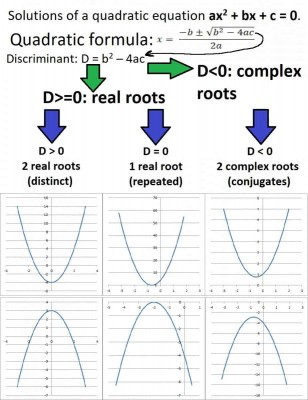A quadratic equation may have two distinct real solutions, one double real (repeated) solution, or two complex conjugate solutions, depending on the sign of the discriminant.

### What Does Quadratic Mean?

Quadratic means that an equation (or function) has a term with the second power of a variable, but no higher order term.  So, there are no variables raised to a third, fourth, or higher power.

Quadratic comes from the Latin “quadratus” meaning square.  This refers to a squared term, which has the second power of a variable.Quadratic comes from the Latin word “quadratus” for square. To find the area of a square with side length x, we must find the value of x*x = x2.

### Examples Of Quadratic Equations

Here are some examples of various quadratic equations, some of which have zero coefficients for b or c (or both).

#### Example 1: A Quadratic Equation With All Nonzero Coefficients

Consider the following quadratic equation:

• 3x2 + 2x + 5 = 0

It has coefficients of a = 3, b = 2, and c = 5 (all of which are nonzero).  The graph of the quadratic is shown below.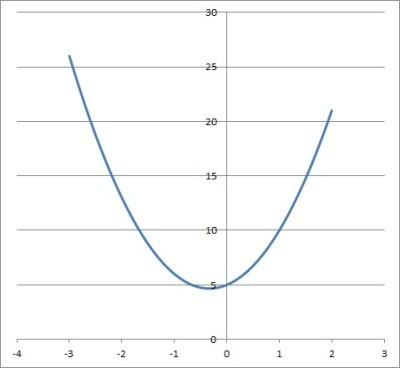The graph of the quadratic function 3x2 + 2x + 5. It turns out that this graph never intersects the x-axis, so it has no real zeros.

#### Example 2: A Quadratic Equation With A Zero Constant Coefficient (c = 0)

Consider the following quadratic equation:

• 4x2 – 8x  = 0

It has coefficients of a = 4, b = -8, and c = 0 (a quadratic equation can have both zero and negative coefficients).  Its graph is shown below.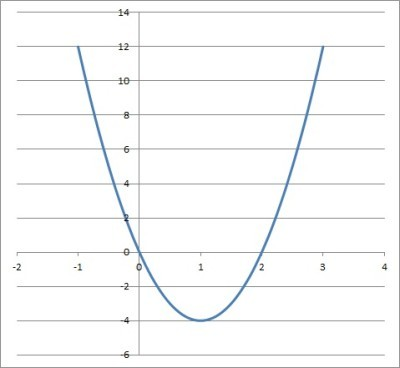The graph of the quadratic function 4x2 – 8x. It turns out that this graph intersects the x-axis at x = 0 and x = 2, so it has two distinct real zeros.

#### Example 3: A Quadratic Equation With A Zero Linear Coefficient (b = 0)

Consider the following quadratic equation:

• -5x2 + 7  = 0

It has coefficients of a = -5, b = 0, and c = 7 (a quadratic equation can have both zero and negative coefficients).  Its graph is shown below.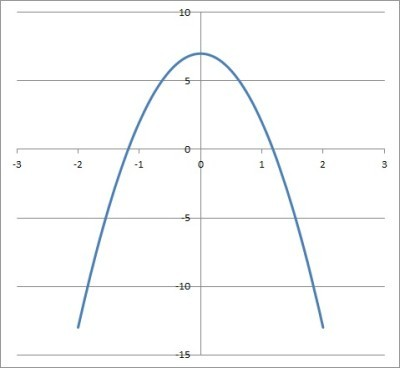The graph of the quadratic function -5x2 + 7. It turns out that this graph intersects the x-axis at two distinct (irrational) points, so it has two distinct real zeros.

#### Example 4: A Quadratic Equation With Zero Linear & Zero Constant Coefficients (b = 0 & c = 0)

Consider the following quadratic equation:

• 10x2 = 0

It has coefficients of a = 10, b = 0, and c = 0 (a quadratic equation can have both the linear and constant coefficients equal to zero, but the quadratic coefficient can never equal zero).  Its graph is shown below.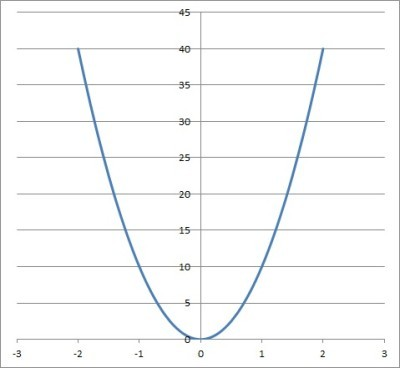The graph of the quadratic function10x2. It turns out that this graph intersects the x-axis at x = 0, so it has one double (repeated) real zero.

## How Can You Tell If An Equation Is Quadratic?

To tell if an equation is quadratic, look at the powers of the variables in the equation.  You should only see powers of 2 (quadratic terms), 1 (linear terms), or 0 (constant terms) in the equation.

If you see any of the following, the equation is not quadratic:

• A “missing” quadratic term (a coefficient of zero for x2, or a = 0).
• a power that is a fraction or decimal (that is not equal to a whole number)
• a power that is negative
• a whole number power that is greater than 2

Let’s look at some examples.

### Example 1: An Equation That Is Not A Quadratic (A Coefficient Of a = 0)

Consider the following equation:

• 4x + 9  = 0

It has a coefficient of a = 0 for the quadratic term.  By definition, this means that it is not a quadratic equation (the first rule above is broken).

The graph is shown below.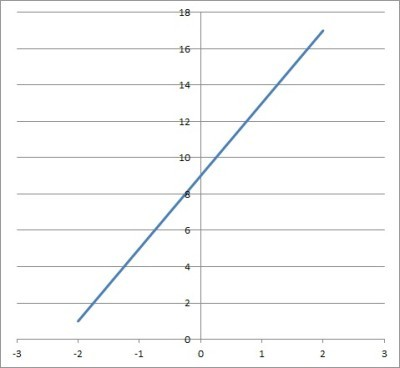The graph of the linear function f(x) = 4x + 9. It has one real zero at x = -2.25. It is not quadratic, since the quadratic coefficient is zero (a = 0).

### Example 2: An Equation That Is Not A Quadratic (A Coefficient That Is A Fraction)

Consider the following equation:

• x2 + 2x – x1/2 + 4 = 0

There is a power of 1/2 in the third term.  Since this is not a whole number, we know that this equation is not quadratic (the second rule above is broken).

The graph is shown below.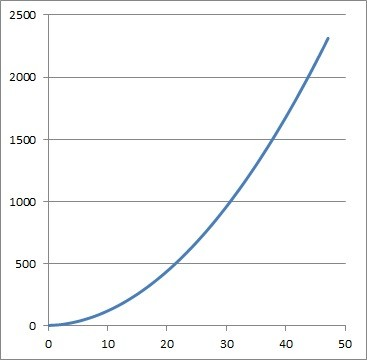The graph of the function x2 + 2x – x1/2 + 4 is not a quadratic, since there is a term with a power of 1/2 for x. The graph looks like a quadratic (where it is defined for x >= 0).

### Example 3: An Equation That Is Not A Quadratic (A Coefficient That Is Negative)

Consider the following equation:

• x2 + 2x-3 + 4 = 0

There is a power of -3 in the second term.  Since this is a negative number, we know that this equation is not quadratic (the third rule above is broken).

The graph is shown below.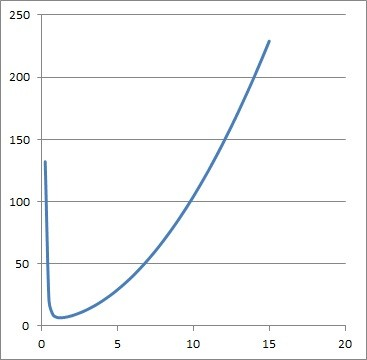The graph of the function x2 + 2x-3 + 4 is not a quadratic, since there is a term with a power of -3 for x. The graph is undefined at x = 0.

### Example 4: An Equation That Is Not A Quadratic (A Coefficient That Is Greater Than 2)

Consider the following equation:

• x4 + 2x2 – 4x + 5 = 0

There is a power of 4 in the second term.  Since this is a whole number greater than 2, we know that this equation is not quadratic (the fourth rule above is broken).

The graph is shown below.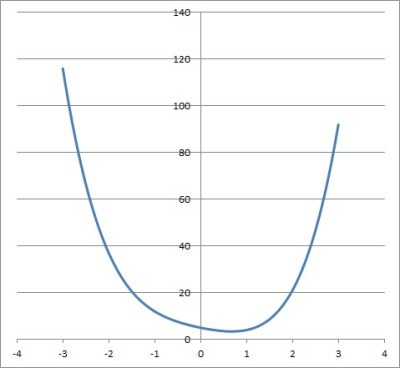The graph of the function x4 + 2x2 – 4x +5 is not a quadratic, since there is a term with a power of 4 for x.

## What Do The Solutions Of A Quadratic Equation Represent?

The solutions of a quadratic equation represent x-values (inputs) where the equation is true.  We can easily factor a quadratic equation in standard form with these solutions (see quadratic factored form above).

Visually, the solutions of a quadratic equation represent the points where a parabola intersects the x-axis (that is, where y = 0).  These are also called zeros of the corresponding quadratic function f(x) = ax2 + bx + c.

## How Do You Solve A Quadratic Equation?

There are four methods you can use to solve a quadratic equation:

• Graphing – this is a good visual method.  If you graph the quadratic function f(x) = ax2 + bx + c, you can find out where it intersects the x-axis.  The only drawback is that it can be difficult to find exact values of x.  Also, the graph will not intersect the x-axis if the solutions are complex (in the case of a negative discriminant).
• Factoring – this method is helpful in some cases to avoid the work of graphing, completing the square, or using the quadratic formula.  However, some quadratics are difficult to factor, and the quadratic formula would be more helpful in those cases.
• Complete the Square – this method is a long one, but it works.  The quadratic formula is a shortcut for this method.  We also use the method of completing the square to put certain circle equations into the proper form.
• Quadratic Formula – this method always works.  It is derived from using the previous method (complete the square) on a general quadratic in standard form: ax2 + bx + c = 0.  The only drawback is that the calculations can become tedious, as they involve multiplication, addition, radicals, and division (fractions).

Sometimes, it is easy to get confused about the difference between a quadratic equation and the quadratic formula.  Here are two key things to remember:

• there are many quadratic equations, but there is only one quadratic formula (it is unique!)
• the quadratic formula is used to solve a quadratic equation

A quadratic equation has the standard form ax2 + bx + c = 0, and it can have two real solutions, one real repeated solution, or two complex conjugate solutions (depending on the sign of the discriminant, as we outlined earlier).

The quadratic formula uses the coefficients a, b, and c from the quadratic equation to help us find these solutions.

### When Do You Use The Quadratic Formula?

You use the quadratic formula to find the zeros (x-intercepts) of a parabola (the shape that a quadratic function takes when graphed).  Knowing the zeros of a function makes it easier to graph correctly.

You can also use the quadratic formula to find the roots of a quadratic equation if factoring is difficult.  For example, the quadratic equation 2x2 + 3x + 9 will not factor easily.

In addition, the quadratic formula is useful in physics to deal with gravity and falling objects.  For example, we can use it to find out when an object fired into the air achieves a certain height (possibly twice: once on the way up, and once again on the way back down).

## What Is A Quadratic Function?

A quadratic function is a function whose standard form has the equation f(x) = ax2 + bx + c, where a is not zero.

If you graph a quadratic function, you get the shape of a parabola.  A quadratic function can have two distinct real zeroes, one repeated real zero, or two complex zeroes (these are points where the parabola intersects the x-axis, or the line y = 0).

When we set a quadratic function equal to zero (solve for y = 0), we get a quadratic equation in standard form: ax2 + bx + c = 0.

## Conclusion

Now you know what a quadratic equation is, what one looks like, and how to solve it.  You also know what the solutions mean.

You can learn about the difference between expressions and equations here.

You can also learn about the differences between linear and quadratic functions here.

I hope you found this article helpful.  If so, please share it with someone who can use the information.

~Jonathon